# Calculus 2 : Comparing Series

## Example Questions

1 3 Next →

### Example Question #2956 : Calculus Ii

Test for convergence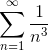Converges absolutely

Cannot be determined

Converges in an interval

Converges conditionally

Diverges

Converges absolutely

Explanation:

Step 1: Recall the convergence rule of the power series:

According to the convergence rule of the power series....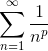converges as long as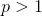Step 2: Compare the exponent:

Since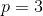, it is greater than. Hence the series converges.

Step 3: Conclusion of the convergence rule

Now, notice that the series isn't an alternating series, so it doesn't matter whether we check for absolute or conditional convergence.

### Example Question #2957 : Calculus Ii

Test for convergence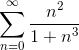Converges absolutely

Converges in an interval

Can't be determined

Converges Conditionally

Diverges

Converges absolutely

Explanation:

Step 1: Try and look for another function that is similar to the original function: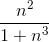looks like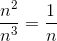Step 2: We will now Use the Limit Comparison test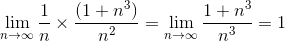Since the limit calculated, is not equal to 0, the given series converges by limit comparison test

### Example Question #2958 : Calculus Ii

Does the following series converge or diverge?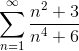Diverge

Conditionally converge

The series either absolutely converges, conditionally converges, or diverges.

Absolutely converge

Absolutely converge

Explanation:

The best way to answer this question would be by comparing the series to another series,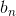, that greatly resembles the behavior of the original series,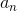. The behavior is determined by the terms of the numerator and the denominator that approach infinity at the quickest rate. In this case: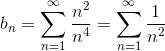When this series is simplifies, it simplifies to a series that converges because of the p-test where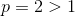.

With two series and the confirmed convergence of one of those series, the limit comparison test can be applied to test for the convergence or divergence of the original series. The limit comparison test states that two series will converge or diverge together if: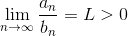Specifically: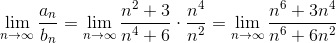This limit equals one because of the fact that: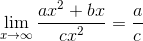if the coefficients come from the same power.

Because the limit is larger than zero,andwill converge or diverge together. Since it was already established thatconverges, the original seies,, converges by the limit comparison test.

### Example Question #2959 : Calculus Ii

Determine if the following series converges or diverges: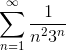Series diverges

Series converges

Series converges

Explanation: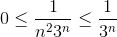for all;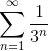is a sum of geometric sequence with base 1/3.

Therefore, said sum converges.

Then, by comparison test,also converges.

### Example Question #2960 : Calculus Ii

What can be said about the convergence of the series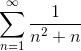?

Diverges

Converges

Inconclusive

Converges

Explanation:

Since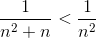for all n>0, and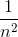converges as a P-Series, we may conclude that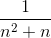must also converge by the comparison test.

### Example Question #171 : Series In Calculus

If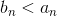and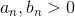, and it may be said that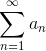converges, what may be said about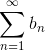?Converges by the ratio test.Diverges by the ratio test.Diverges by the comparison test.Converges by the comparison test.Converges by the test for convergence of geometric series.Converges by the comparison test.

Explanation:

Given two series,andwhereconverges,

the Comparison Test states that the second seriesmust also converge, if and only if, it is smaller than the first.

1 3 Next →